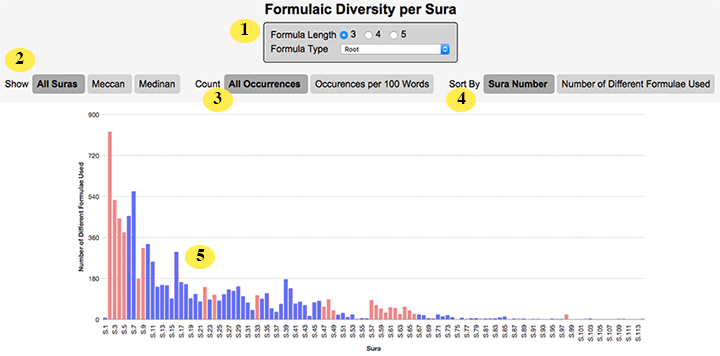Qur’an Tools is not optimised for narrow screens

The Formulaic Diversity per Sura chart (found under the ‘Charts’ menu and then under ‘Formulae’) shows you how many different formulae are used in each sura. (Find out more about formulaic phraseology, how to study it in Qur’an Tools, and some of the implications of the Qur’an’s heavy use of formulae here).1 Formula Type and Length to Show. This allows you to customise the type of formulae that Qur’an Tools counts and shows in the chart below. (The length is the number of Arabic words in a formula; the type allows you to choose from any of the three formula types that Qur’an Tools understands, or to choose ‘All Formula Types’ which means the chart will count what percentage of the words in each sura are part of any type of formula. 2 Suras to Show. By clicking the buttons, you can choose whether to plot every sura, just Meccan suras, or just Medinan suras on the chart. 3 Count. By clicking the buttons, you can choose whether to count every formulae in each sura, or instead count how many formulae occur per 100 words. (The latter is useful as it helps adjust for the fact that some suras are much longer than others, so you can see the relative formulae use per sura). 4 Sort By. You can either sort your chart by sura (so sura 1 is drawn first and sura 114 last), or you can sort your chart by the number of different formula (meaning suras with a greater number of different formulae in them will appear first). 5 Chart Columns. Point your mouse at a column and Qur’an Tools will show you a tooltip with the exact value in it. You can also click a column to “drill down” — Qur’an Tools will show you a list of every formulae in the sura whose column you clicked.

TIP

This chart counts the number of different formulae in a sura (e.g. if a sura used one formulae ten times, it would show a diversity of "1" on this chart). If you’d prefer to count how many formulae in total occur in each sura, you’d want to use the ‘Number of Formulae Used per Sura’ chart instead.